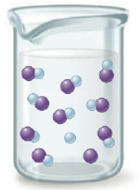# The following illustration displays the relative number of species when an acid, HA, is added to water. a. Is HA a weak or strong acid? How can you tell? b. Using the relative numbers given in the illustration, determine the value for K a and the percent dissociation of the acid. Assume the initial acid concentration is 0.20 M.### Chemistry: An Atoms First Approach

2nd Edition
Steven S. Zumdahl + 1 other
Publisher: Cengage Learning
ISBN: 9781305079243

#### Solutions

Chapter
Section### Chemistry: An Atoms First Approach

2nd Edition
Steven S. Zumdahl + 1 other
Publisher: Cengage Learning
ISBN: 9781305079243
Chapter 13, Problem 153AE
Textbook Problem
1 views

## The following illustration displays the relative number of species when an acid, HA, is added to water.a. Is HA a weak or strong acid? How can you tell?b. Using the relative numbers given in the illustration, determine the value for Ka and the percent dissociation of the acid. Assume the initial acid concentration is 0.20 M.

(a)

Interpretation Introduction

Interpretation: The given acid HA strong or weak, value of Ka for HA upon addition to water, percentage dissociation of HA is to be stated.

Concept introduction: Strong acids dissociate completely and rapidly when dissolved in an aqueous solution and has very large value of Ka while the weak acids dissociates partially and slowly in aqueous solution and has very low value of Ka .

Strong acids have high value of percentage dissociation while weak acids have low value of percentage dissociation.

To determine: The given acid HA is strong or weak.

### Explanation of Solution

Explanation

The given illustration is,

Figure 1

The given acid HA

(b)

Interpretation Introduction

Interpretation: The given acid HA strong or weak, value of Ka for HA upon addition to water, percentage dissociation of HA is to be stated.

Concept introduction: Strong acids dissociate completely and rapidly when dissolved in an aqueous solution and has very large value of Ka while the weak acids dissociates partially and slowly in aqueous solution and has very low value of Ka .

Strong acids have high value of percentage dissociation while weak acids have low value of percentage dissociation.

To determine: The value of Ka for HA upon addition to water, percentage dissociation of HA .

### Still sussing out bartleby?

Check out a sample textbook solution.

See a sample solution

#### The Solution to Your Study Problems

Bartleby provides explanations to thousands of textbook problems written by our experts, many with advanced degrees!

Get Started

Find more solutions based on key concepts
Fasting hypoglycemia maybe caused by all except a. pancreatic tumors. b. poorly controlled diabetes. c. overuse...

Nutrition: Concepts and Controversies - Standalone book (MindTap Course List)

A ________ is dissolved in a solvent. a. molecule b. salt c. solute d. chemical bond

Biology: The Unity and Diversity of Life (MindTap Course List)

What is the derivation of the word “vaccination”?

Introduction to General, Organic and Biochemistry

How do Newton’s laws lead you to conclude that gravitation has to be universal?

Horizons: Exploring the Universe (MindTap Course List)

A Show that the dot product is commutative: AB=BA (Eq. 9.12).

Physics for Scientists and Engineers: Foundations and Connections

A long, cylindrical conductor of radius R carries a current I as shown in Figure P29.22. The current density J,...

Physics for Scientists and Engineers, Technology Update (No access codes included)